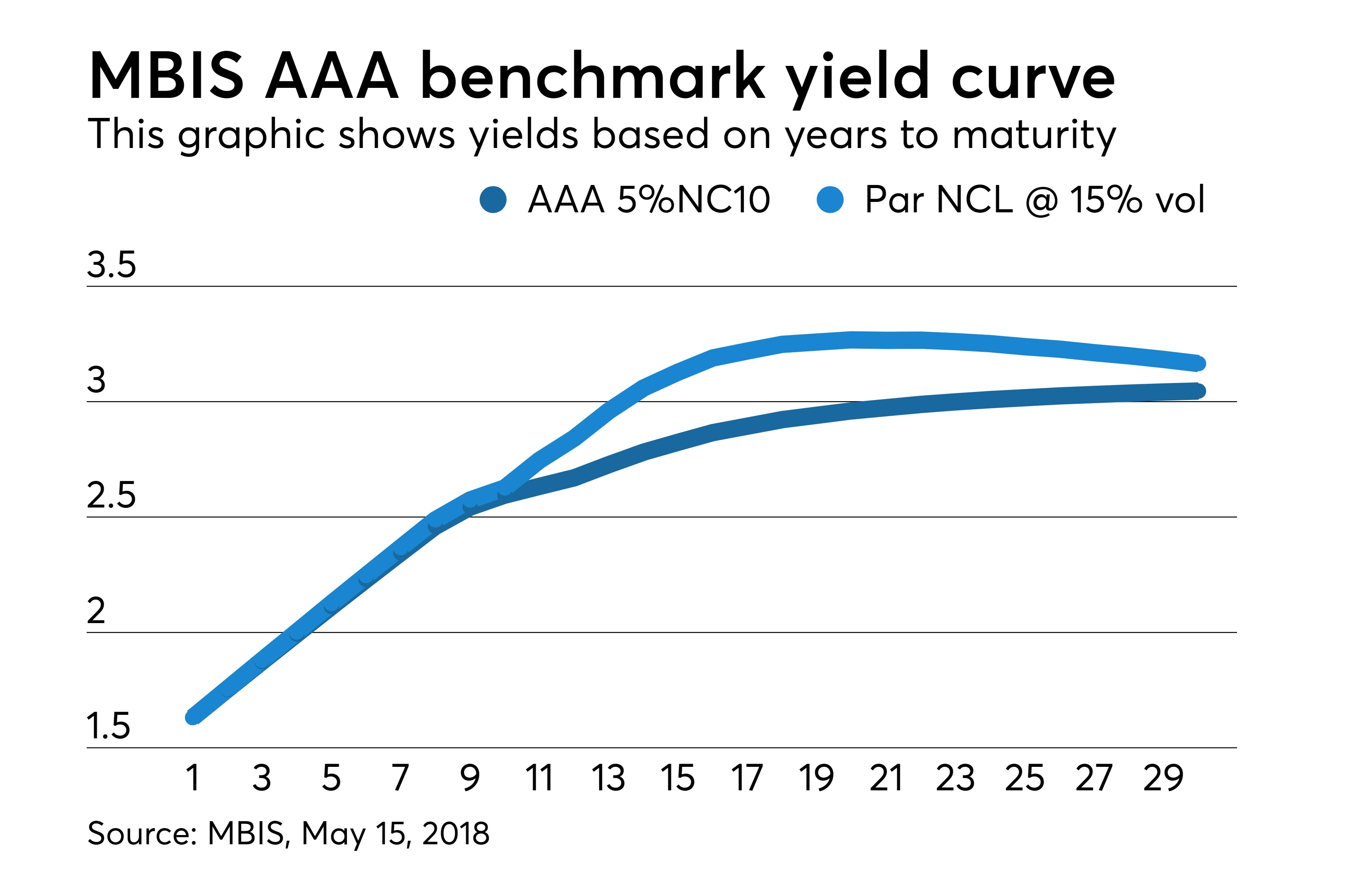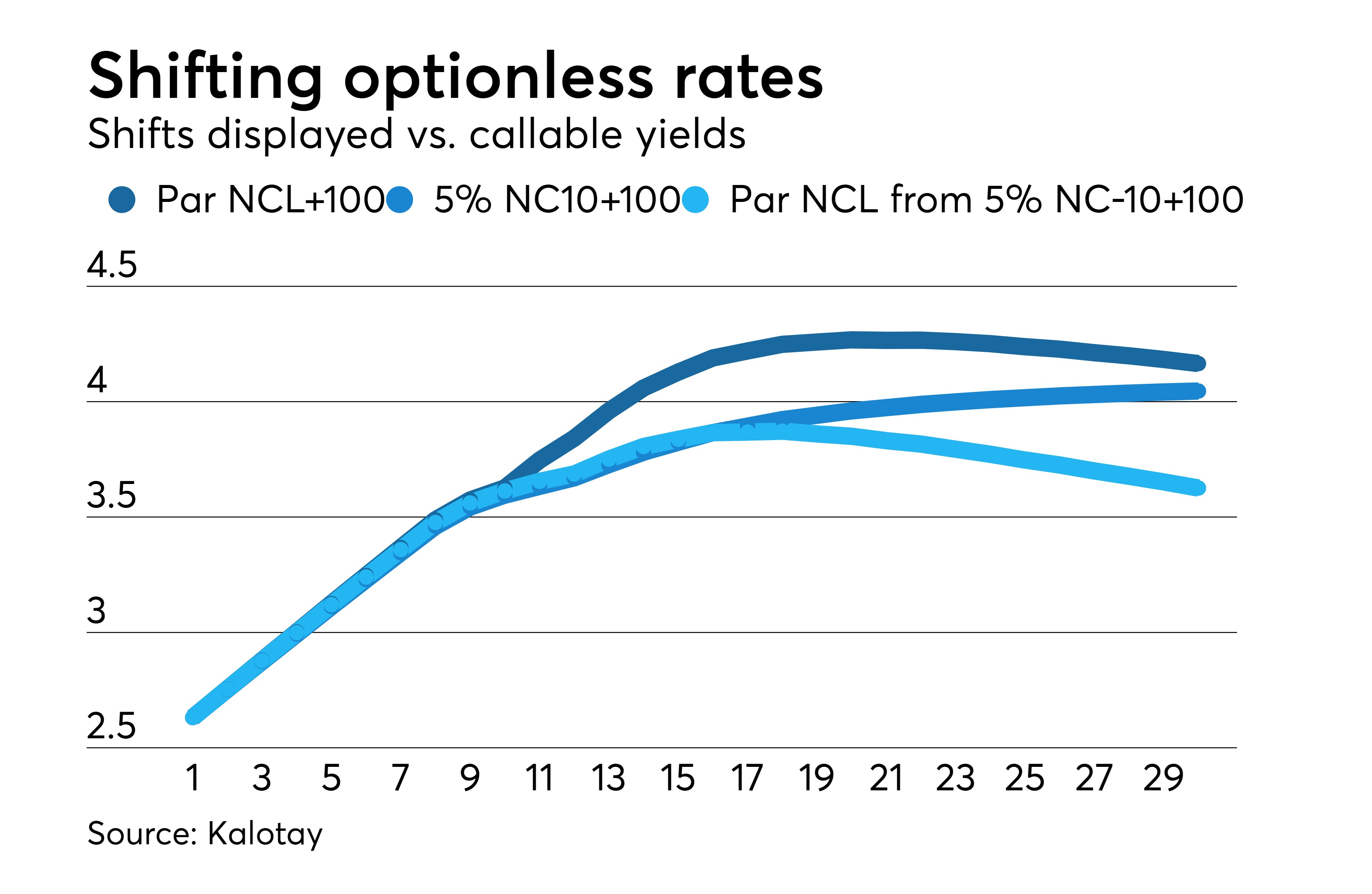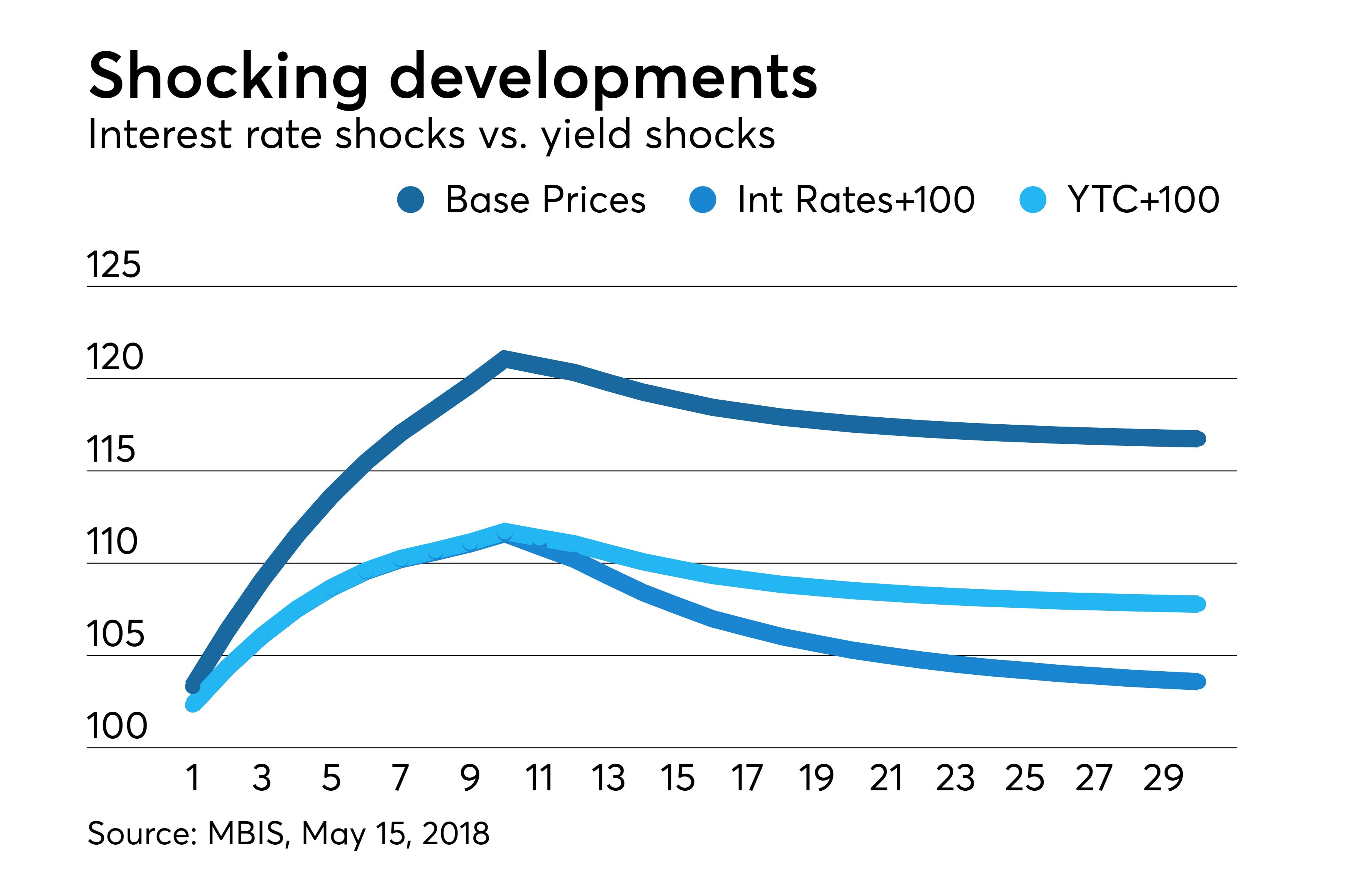# Yield shocks underestimate the interest rate risk of callable bonds

Register now

When thinking of interest rates in the taxable world, practitioners look at bellwether indicators such as the 10-year U.S. Treasury yield, and more broadly at the Treasury yield curve — a representation of the interest rates that investors demand across the maturity spectrum. The frequency of the U.S. Treasury’s issuance of bonds at or near par and the liquidity of that market make the Treasury yield curve an ideal benchmark.

When bonds are issued as callable in 10 years with above-market 5% coupons, as is the current practice with munis, equating yields and interest rates becomes questionable. Muni benchmark yield curves represent the yields-to-call of these bonds. Thinking of the yield-to-call of, say, the 5% 20-year benchmark bond as the 20-year muni interest rate has distorting consequences.

Stress Testing
Why belabor the distinction between interest rates and yields? Because practitioners want to know what happens to the value of their portfolios if interest rates change. As we’ll see, for munis, shocking yields, instead of interest rates, can produce wildly misleading results. Inconveniently, long muni interest rates are not directly observable. However, they can be estimated from long callable yields by stripping out the call option, using the standard option-adjusted spread (OAS) approach.

Figure 1 shows recent MBIS 5% NC-10 AAA benchmark yields, available on the Bond Buyer Data Workstation. The implied optionless (non-call life or NCL) par interest rates, extracted by stripping out the call option assuming a 15% interest rate volatility. For example, the 20-year yield-to-call is 2.960%, while the implied 20-year interest rate is 3.268%A shift of yields implies a corresponding shift of interest rates. As shown in Figure 2, shifting the yields up 100 bps implies the interest rates shown in red. Figure 2 also displays the result of shifting the original interest rates (from Figure 1) by 100 bps. Clearly, shifting yields produces a much smaller shift of interest rates than expected. Clearly, interest rates moved much less than 100 bps. For example, the 30-year interest rate moved from 3.165% to 3.626% — a mere 46 bps.Where the Rubber Hits the Road
The consequences for stress testing or horizon analysis can be stark. To keep it simple, pretend you own 5% NC-10 bonds whose yields coincide with those of the MBIS AAA benchmark curve.

Figure 3 displays the prices of the benchmark bonds corresponding to a 100 bps increase of yields and to a like increase of interest rates. The latter prices were calculated using standard OAS methodology at 15% volatility. As we see, the prices from interest rate shifts decline much more than from yield shifts. For example, shifting interest rates reduces the price of the 30-year bond from 116.74 to 103.58. Merely shifting the 30-year yield-to-call reduces the price to 107.78 — an underestimation of the potential damage by about 4 points!The takeaway from Figure 3 is that shifting yields works well enough for optionless bonds (maturities up to 10 years), but falls apart for callable bonds. You can run but you can’t hide from OAS. The option-adjusted duration of the 30-year benchmark bond today is around 11 years (a 1% rise in rates would result in an 11% decline in price). On the other hand, the price change corresponding to shifting the yield-to-call of any 5% NC-10 bond is reflective of the duration of a 10-year 5% optionless bond, about 8 years, because yield-to-call discounts flows only to the call date. So the price of the 30-year benchmark declines roughly 8%, as does the price of every other callable benchmark!

Bottom line: When it comes to callable bonds, stress testing with yield shocks can severely underestimate the interest rate risk of your portfolio. For the correct approach, see “Why OAS is the Right Tool for Stress Testing.